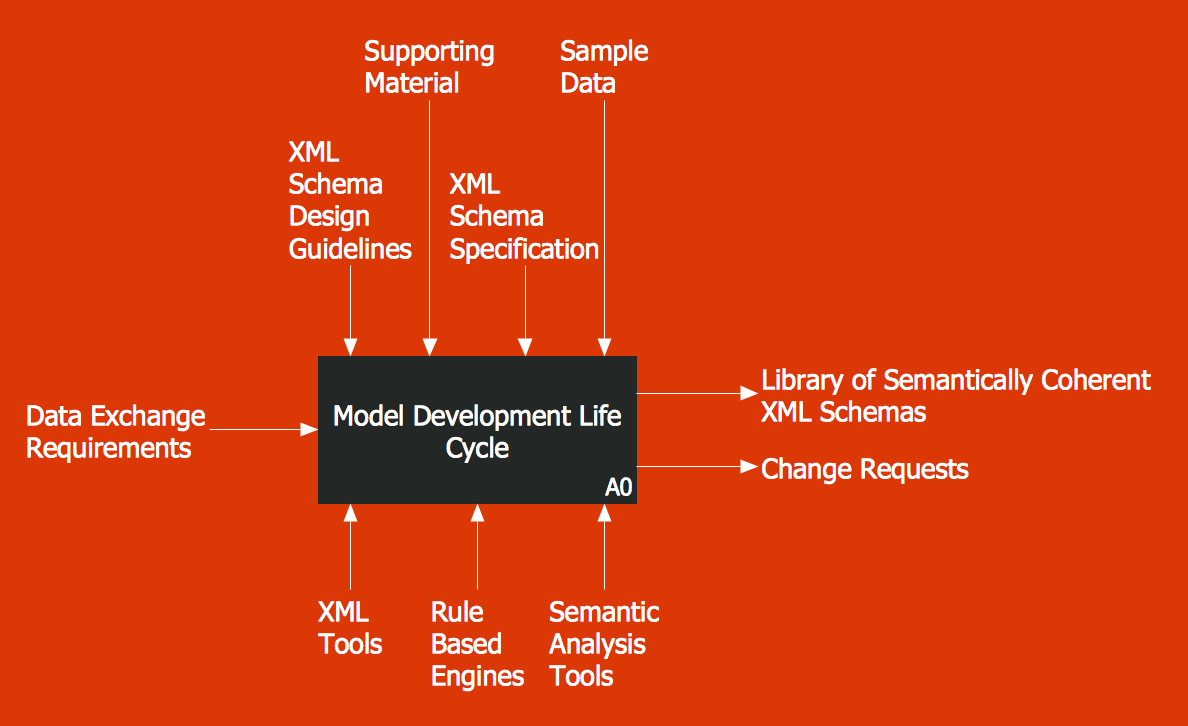This site uses cookies. By continuing to browse the ConceptDraw site you are agreeing to our Use of Site Cookies.
ConceptDraw Samples | Software development — IDEF0 diagrams

# IDEF0 Diagrams

The IDEF0 diagrams are created using ConceptDraw DIAGRAM diagramming and vector drawing software enhanced with IDEF0 Diagrams solution from ConceptDraw Solution Park.

ConceptDraw DIAGRAM provides export of vector graphic multipage documents into multiple file formats: vector graphics (SVG, EMF, EPS), bitmap graphics (PNG, JPEG, GIF, BMP, TIFF), web documents (HTML, PDF), PowerPoint presentations (PPT), Adobe Flash (SWF).

## Tutorials and Solutions:

Video Tutorials: ConceptDraw Solution Park

Solutions: IDEF0 Diagrams for ConceptDraw DIAGRAM

## Sample 1: IDEF0 Diagram — Application Development

IDEF0 Diagrams sample: Application development.

This example is created using ConceptDraw DIAGRAM diagramming software enhanced with IDEF0 Diagrams solution from ConceptDraw Solution Park.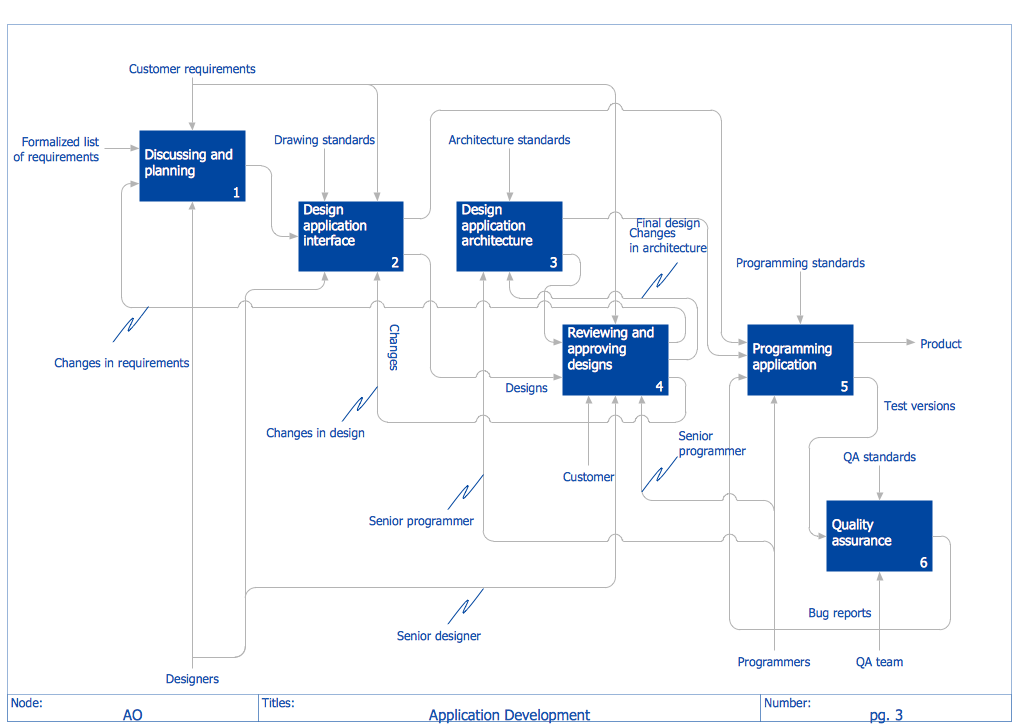## Sample 2: IDEF0 Diagram — Model Integration

IDEF0 Diagrams sample: Model integration.

This example is created using ConceptDraw DIAGRAM diagramming software enhanced with IDEF0 Diagrams solution from ConceptDraw Solution Park.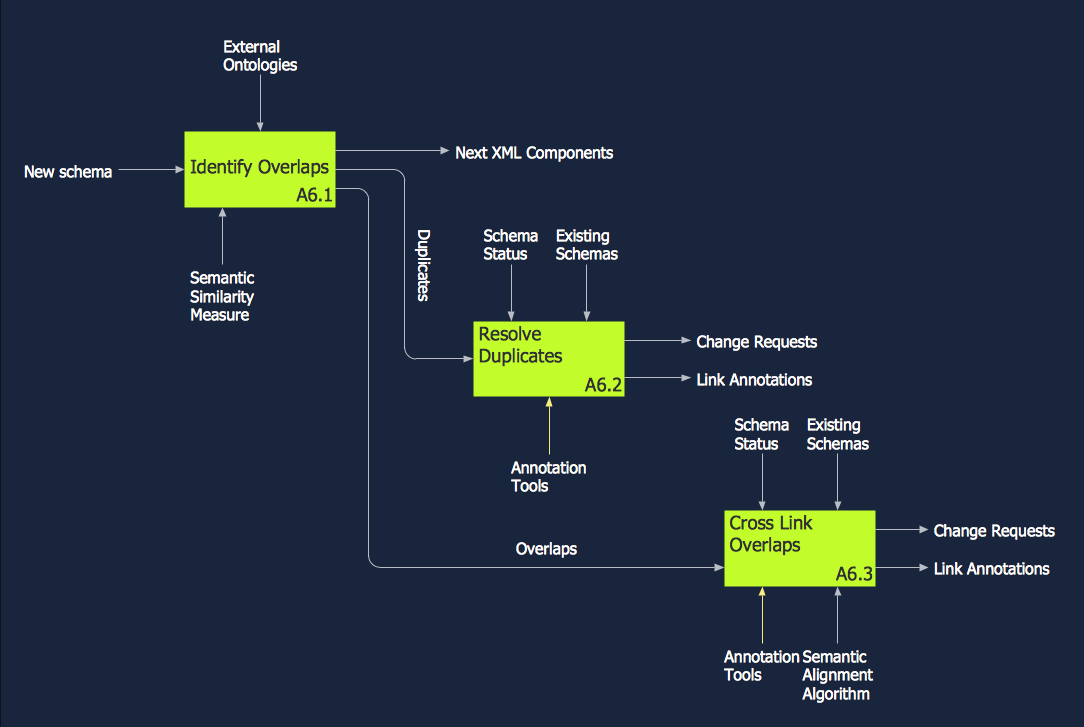## Sample 3: IDEF0 Diagram — Top Level Context Diagram

IDEF0 Diagrams sample: Top level context diagram.

This example is created using ConceptDraw DIAGRAM diagramming software enhanced with IDEF0 Diagrams solution from ConceptDraw Solution Park.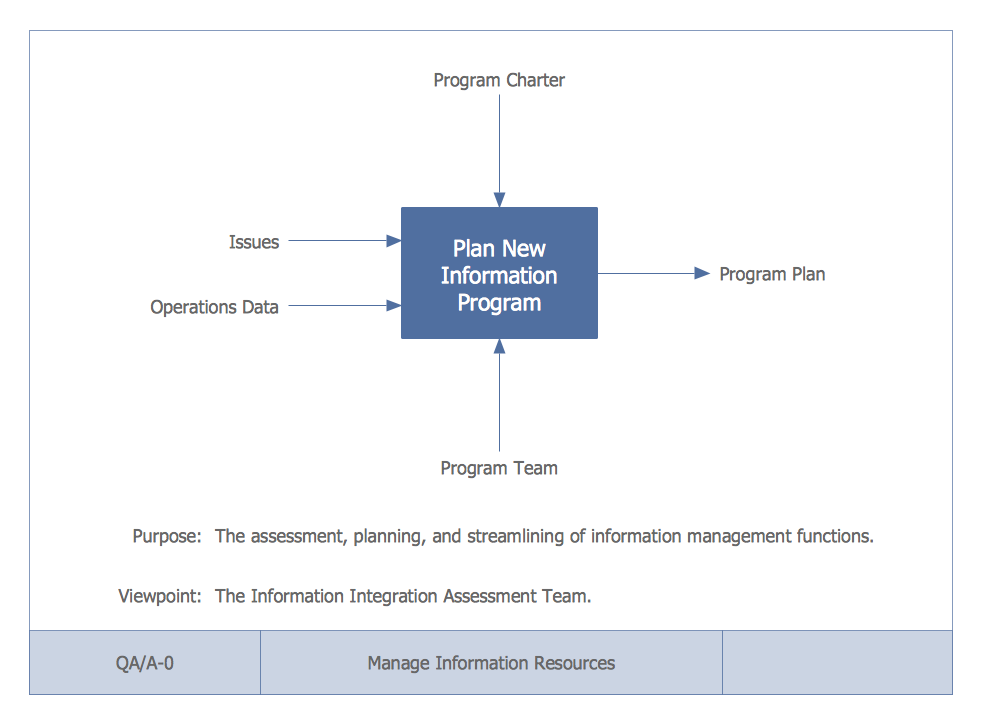## Sample 4: IDEF0 Diagram — Determine Groundwater

IDEF0 Diagrams sample: Determine groundwater.

This example is created using ConceptDraw DIAGRAM diagramming software enhanced with IDEF0 Diagrams solution from ConceptDraw Solution Park.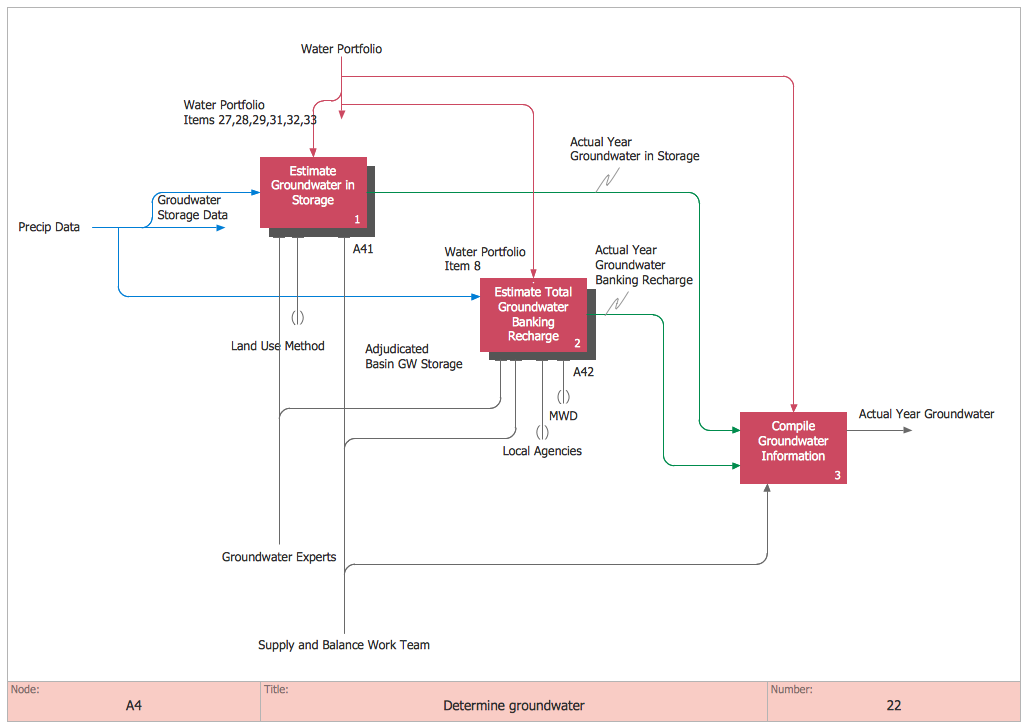## Sample 5: IDEF0 Diagram — Model Development Life Cycle

IDEF0 Diagrams sample: Model development life cycle.

This example is created using ConceptDraw DIAGRAM diagramming software enhanced with IDEF0 Diagrams solution from ConceptDraw Solution Park.